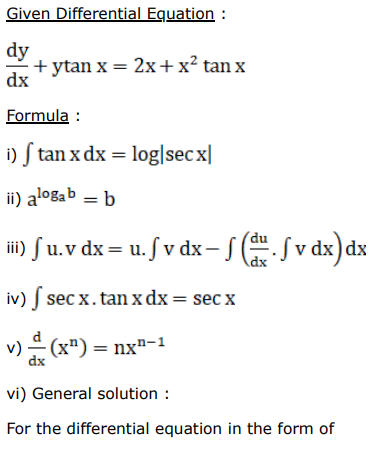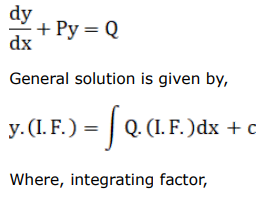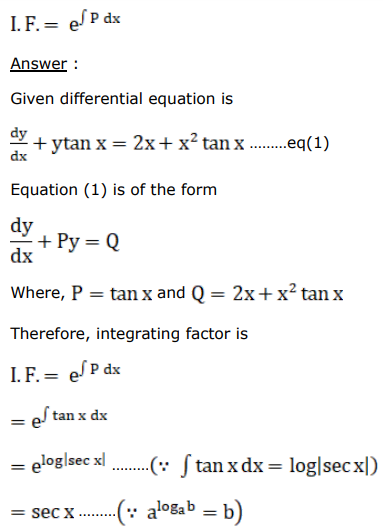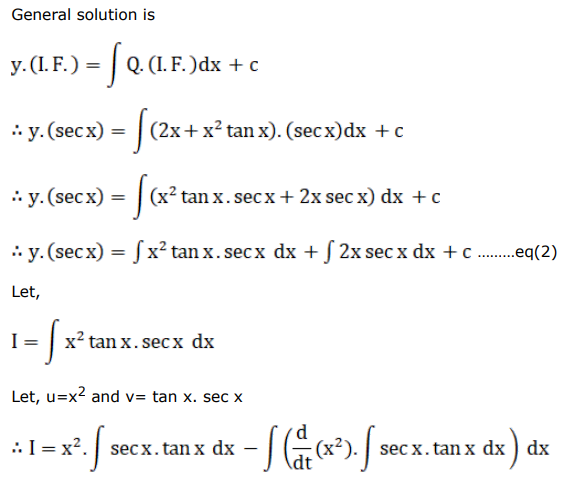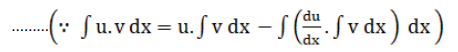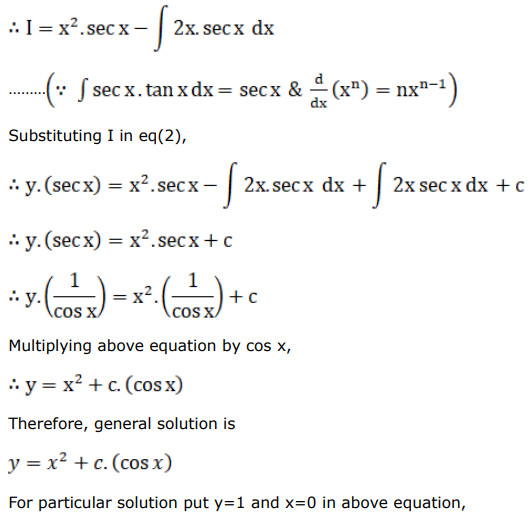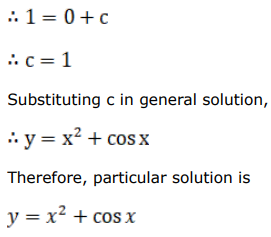# Find a particular solution satisfying the given

Question:

Find a particular solution satisfying the given condition for each of the following differential equations.

$\frac{d y}{d x}+y \tan x=2 x+x^{2} \tan x$, given that $y=1$ when $x=0$

Solution: